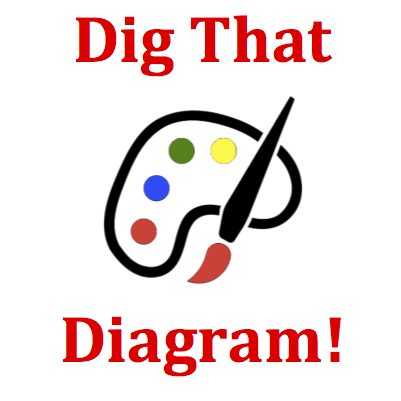# Circular and Satellite Motion - Mission CG2 Detailed HelpAn object is moving in a circle at a constant speed in a 'clockwise fashion.' A 'God's eye' view of the circle is shown. For the indicated position, the direction of the velocity vector is represented by arrow ____; the direction of the acceleration vector is represented by ____; and the direction of the net force vector is represented by arrow ____.The diagram uses a large dot to represent a specific location along a circle about which an object is moving. You are to pick three arrows that represent the directions of the velocity, acceleration and net force vectors at this location. Use the information in the Know the Law sections to complete this question.Uniform Circular Motion and Velocity: Velocity is a vector and has a direction. The direction of an object's velocity is always in the same direction that the object is moving. For an object moving in a circle at constant speed, the velocity vector is always directed in a direction that is tangent to the circle.Uniform Circular Motion and Acceleration: Accelerating objects are changing their velocity - either the magnitude or the direction of the velocity. Objects moving in a circle at a constant speed are accelerating due to their changing direction. The direction of the acceleration is directed toward the center of the circle about which the object moves. Such a direction is described as being centripetal.Uniform Circular Motion and Net Force: An object which moves in a circle is accelerating. Accelerations are caused by an unbalanced or net force. The net force is always in the same direction as the acceleration. For objects moving in circles at constant speed, the net force is directed towards the center of the circle about which the object moves. Such a direction is described as being centripetal.